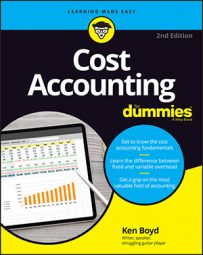##### Cost Accounting For DummiesIn cost accounting, the cost of goods available for sale represents the product’s total costs. Total costs have two components — joint costs and separable costs.

Assume the cost of goods available for sale are \$1,751,163 and \$1,260,837 for the heavy-duty blower and the yardwork blower. Say the separable costs are \$1,200,000 and \$912,000. If you know the separable costs and the cost of goods available for sale, you can compute the joint cost allocation. The first table shows the process.

Joint Cost Allocation
Heavy-Duty Yardwork Total
Cost of goods available for sale \$1,751,163 \$1,260,837 \$3,012,000
Less separable costs \$1,200,000 \$912,000 \$2,112,000
Equals joint cost allocation \$551,163 \$348,837 \$900,000
Each company division provides the separable costs. So altogether, the table gives you a joint cost allocation.

Now calculate the gross margin percentage. Say your sales values are \$2,000,00 and \$1,440,000 for heavy-duty and yardwork blowers. The total cost is the cost of goods available for sale from the first table. The gross margin percentage is the gross margin divided by the sales value. For each product, the gross margin percentage is the same (12.442 percent) as the company’s overall gross margin.

Verifying Gross Margin Percentage
Heavy-Duty Yardwork Total
Sales value (A) \$2,000,000 \$1,440,000 \$3,440,000
Total cost (B) \$1,751,163 \$1,260,837 \$3,012,000
Gross margin (A B) \$248,837 \$179,163 \$428,000
Gross margin percentage 12.442 12.442
Here’s the point of this table: it uses the traditional formula to compute gross margin and gross margin percentage. The table verifies that the calculations are correct.

If the heavy-duty product has the higher sales value, it ends up with a higher gross margin in dollars than the yardwork product. However, both sale values are multiplied by the same gross margin percentage. Both products have a gross margin of about 12.5 percent (rounded). That means that about 87.5 percent of sales value represents cost of goods available for sale.